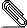# [NTG-context] \setupmathematics: autopunctuation only for certain symbols like . or ,

Dear ConTeXt folks,


if I am not mistaken autopunctuation  should prevent the space
after the decimal mark  like in »1,23« or »1.23«.

The following minimal example, which is also attached, indicates that
the space is also not added for semicolon »;«.

--- 8< --- minimal example --- >8 ---
\starttext
\setupformulas[align=right]

\startformula
ℕ ≔ \left\{ 0; 1; 2; 3; … \right\}
\stopformula

\setupmathematics[autopunctuation=no]
\startformula
ℕ ≔ \left\{ 0; 1; 2; 3; … \right\}
\stopformula
\stoptext
--- 8< --- minimal example --- >8 ---

I wonder if this is on purpose because the Wiki page  does not list
the semicolon as a decimal mark.

Would it be possible to take the semicolon out of the handling of
autopunctuation?

Thanks,

Paul

 http://wiki.contextgarden.net/Math_setups
 https://secure.wikimedia.org/wikipedia/en/wiki/Decimal_markmenge.pdf

\starttext
\setupformulas[align=right]

\startformula
ℕ ≔ \left\{0; 1; 2; 3; … \right\}
\stopformula

\setupmathematics[autopunctuation=no]
\startformula
ℕ ≔ \left\{0; 1; 2; 3; … \right\}
\stopformula
\stoptextsignature.asc
Description: This is a digitally signed message part

___________________________________________________________________________________
___________________________________________________________________________________